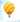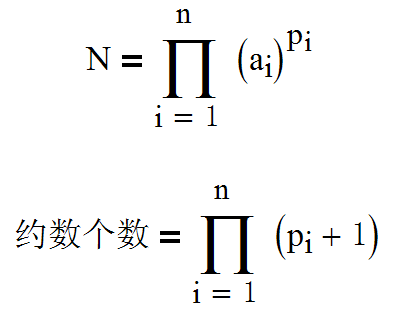## 勇敢的CSDNer

Wed, 29th January 2020Edit on Github多方博弈

2009年6月CSDN中有网友提出了多方博弈问题:
10位CSDNer乘坐热气球在太平洋上空旅行，他们打算开香槟庆祝一下这个伟大的时刻。然而很不幸，他们发现气球上破了一个洞，氢气不断外泄，气球开始下降，很快他们就会掉到海洋里成为鲨鱼的甜品。

1
2
6
1
3
1
1
1
1
1

a的约数有：1，2，3，4，6，9，12，18，36总共9个

shshsh_0510把问题进口到数学研发论坛, 并且询问，CSDNer能否有什么策略让别人更英雄一些吗？

# 详细信息mathe对大家的分析做了更加详细的解释:

KeyTo9_Fans登堂亮相，充分显示了ACMer的能量，进行了大量的模拟,比如:
$41$次迭代，重复$100$万次试验，得到$0$号选手的死亡率已经高达$90.4%$了，

$41$次迭代：

$0$ $0.904004$
$1$ $9.580946\times 10^{-13}$
$2$ $0.024025$
$3$ $1.198199\times 10^{-12}$
$4$ $0.024178$
$5$ $8.687553\times 10^{-10}$
$6$ $0.024050$
$7$ $1.196017\times 10^{-12}$
$8$ $0.023969$
$9$ $1.125888\times 10^{-12}$

【任何$2$个人在其余$8$人都不改变策略的前提下进行$2$人博弈，他们仍然会继续使用自己当前的策略】。
【任何$3$个人在其余$7$人都不改变策略的前提下进行$3$人博弈，他们仍然会继续使用自己当前的策略】。
……
【任何$9$个人在剩余$1$人不改变策略的前提下进行$9$人博弈，他们仍然会继续使用自己当前的策略】。

Github